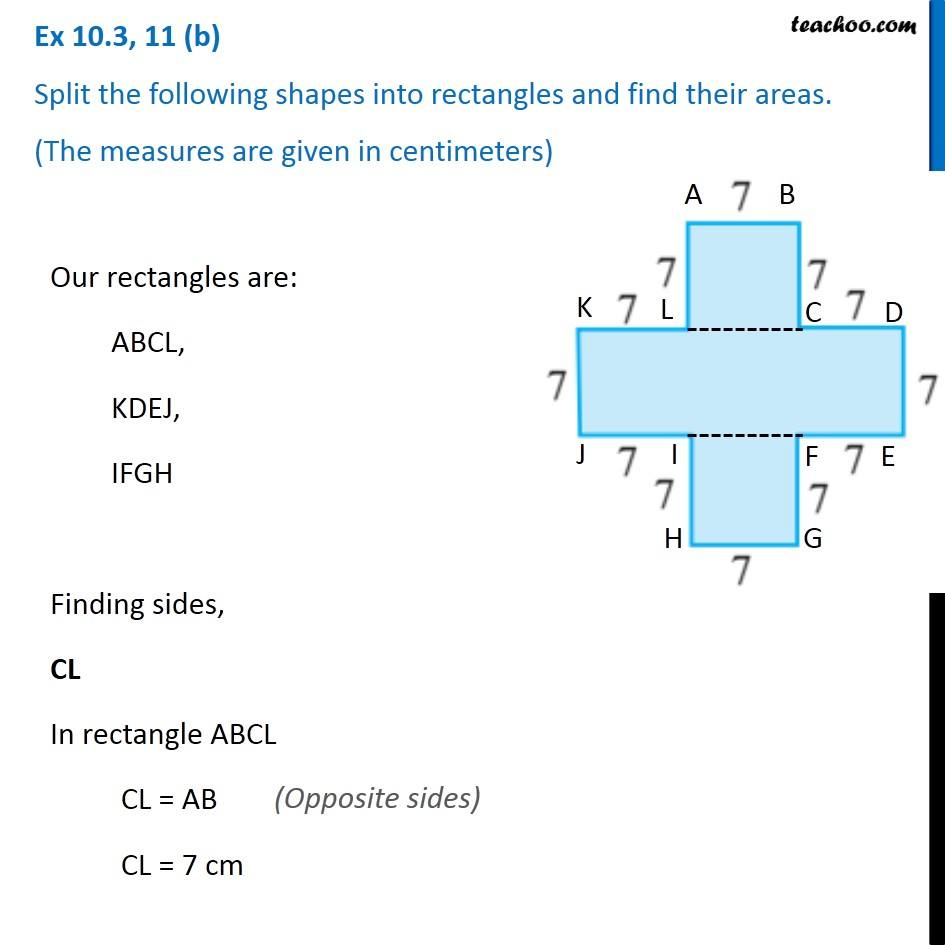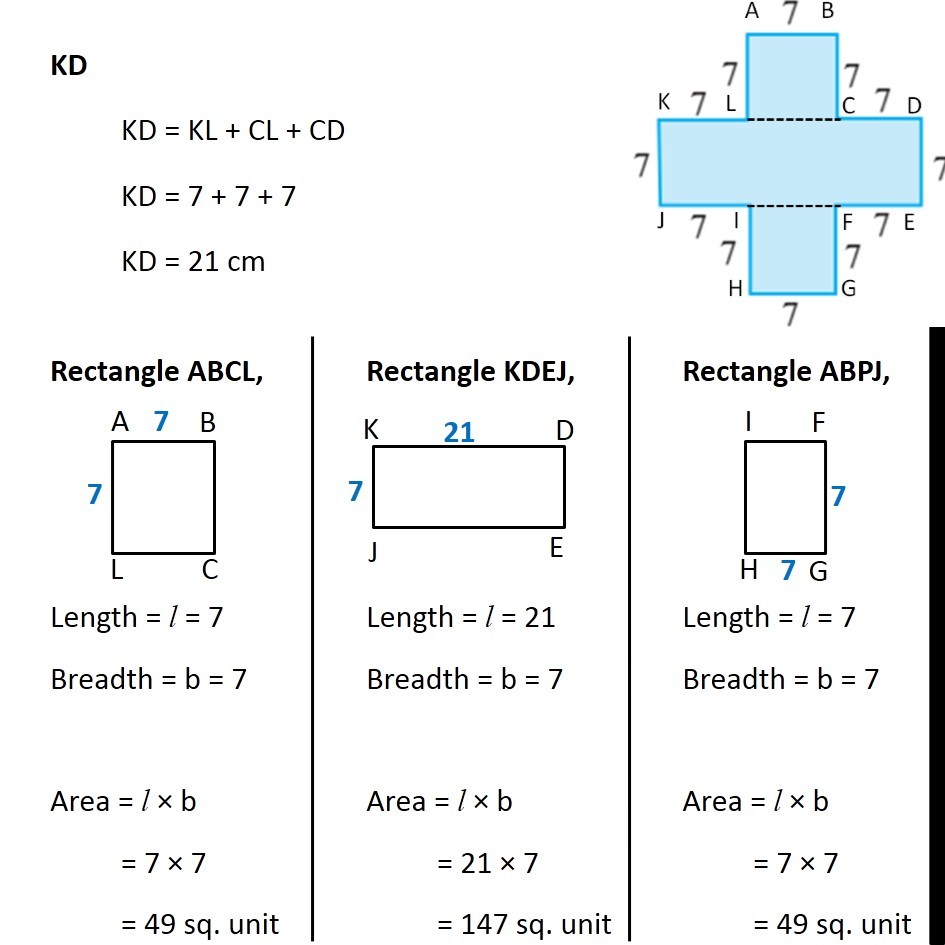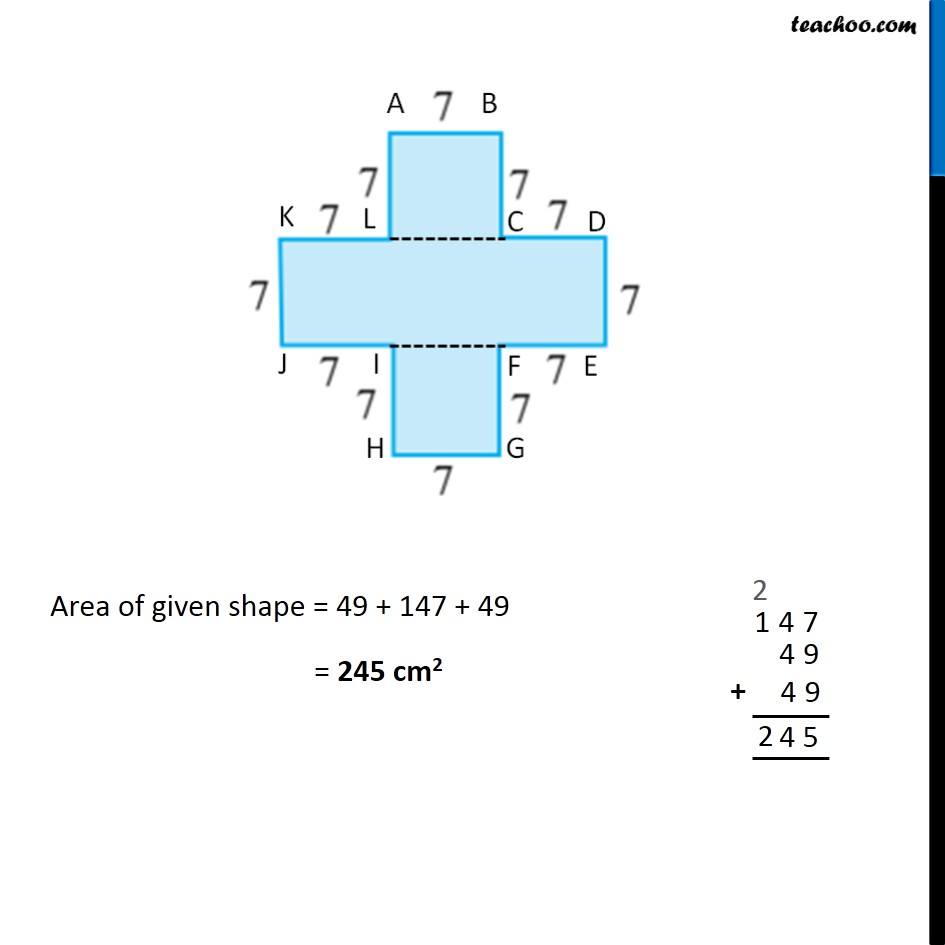Ex 10.3

Chapter 10 Class 6 Mensuration
Serial order wiseLearn in your speed, with individual attention - Teachoo Maths 1-on-1 Class

### Transcript

Ex 10.3, 11 (b) Split the following shapes into rectangles and find their areas. (The measures are given in centimeters) Our rectangles are: ABCL, KDEJ, IFGH Finding sides, CL In rectangle ABCL CL = AB CL = 7 cm (Opposite sides) KD KD = KL + CL + CD KD = 7 + 7 + 7 KD = 21 cm Rectangle ABCL, Length = l = 7 Breadth = b = 7 Area = l × b = 7 × 7 = 49 sq. unit Rectangle KDEJ, Length = l = 21 Breadth = b = 7 Area = l × b = 21 × 7 = 147 sq. unit Rectangle ABPJ, Length = l = 7 Breadth = b = 7 Area = l × b = 7 × 7 = 49 sq. unit Area of given shape = 49 + 147 + 49 = 245 cm2